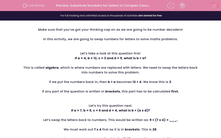# Substitute Numbers for Letters in Complex Calculations

In this worksheet, students will replace letters with numbers and carry out questions using the four number operations, with some calculations in sets of brackets. They must give their final answers as numbers not letters.Key stage:  KS 2

Curriculum topic:   Verbal Reasoning

Curriculum subtopic:   Maths Codes

Difficulty level:#### Worksheet Overview

Make sure that you’ve got your thinking cap on as we are going to be number decoders!

In this activity, we are going to swap numbers for letters to solve maths problems.

Let’s take a look at this question first:

If a = 4, b = 12, c = 3 and d = 9, what is b ÷ a?

This is called algebra, which is where numbers are replaced with letters. We need to swap the letters back into numbers to solve this problem.

If we put the numbers back in, then b ÷ a becomes 12 ÷ 4. We know this is 3.

If any part of the question is written in brackets, this part has to be calculated first.

Let’s try this question next:

If a = 7, b = 9, c = 6 and d = 4, what is b + (a x d)?

Let’s swap the letters back to numbers. This would be written as: 9 + (7 x 4) = ___.

We must work out 7 x 4 first as it is in brackets. This is 28.

Our number sentence now looks like this: 9 + (28) = ___. The answer is 37.

Let’s try one more together:

If a = 8, b = 10, c = 4 and d = 12, what is b (a + c)?

Where there isn’t a -, +, x or ÷ between the first letter and the bracket, it means we have to multiply the outside number by what is inside the brackets. We must imagine an invisible times sign here.

This can be written as: b x (a + c) = ___.

In numbers this is: 10 x (8 + 4) = ____.

We must solve the brackets first: 8 + 4 = 12.

Our number sentence now becomes: 10 x (12) = 120. So our answer is 120.

Now it’s your turn to crack the codes. Good luck number detective!

### What is EdPlace?

We're your National Curriculum aligned online education content provider helping each child succeed in English, maths and science from year 1 to GCSE. With an EdPlace account you’ll be able to track and measure progress, helping each child achieve their best. We build confidence and attainment by personalising each child’s learning at a level that suits them.

Get started